## 0. 前言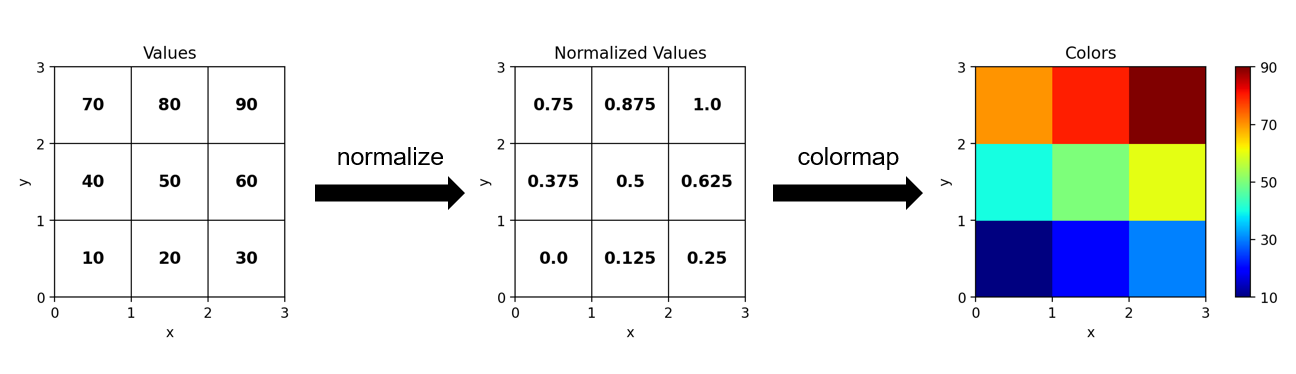• 首先将数组归一化（normalize）到浮点型的 [0, 1] 范围（或整型的 [0, N - 1] 范围）上去。
• 再把归一化的数组输入给 colormap，查询每个数值对应的颜色。

（2022-01-17 更新：增加了一些解释说明，删掉了不实用的介绍，加入了 BoundaryNorm 实现的红蓝色标的例子。）

## 1. Colormap

Colormap 分为两个子类：ListedColormapLinearSegmentedColormap，它们被存放在 matplotlib.colors 模块下。在介绍它们之前先做点准备工作

import numpy as np
import matplotlib.pyplot as plt
import matplotlib.cm as cm
import matplotlib.colors as mcolors

def show_cmap(cmap, norm=None, extend=None):
'''展示一个colormap.'''
if norm is None:
norm = mcolors.Normalize(vmin=0, vmax=cmap.N)
im = cm.ScalarMappable(norm=norm, cmap=cmap)

fig, ax = plt.subplots(figsize=(6, 1))
fig.colorbar(im, cax=ax, orientation='horizontal', extend=extend)
plt.show()


### 1.1 ListedColormap

ListedColormap(colors, name='from_list', N=None)


colors 是颜色名组成的列表或 RGB(A) 数组，nameN 分别是该 colormap 的名字和所含颜色数，不过自定义对象一般不需要取名，颜色数默认为 len(colors)，所以这两个参数基本用不上。这些参数随后会被赋给对象的同名属性。例如

colors = ['darkorange', 'gold', 'lawngreen', 'lightseagreen']
cmap = mcolors.ListedColormap(colors)
show_cmap(cmap)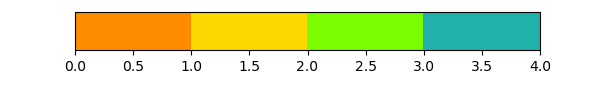In : cmap.colors
Out: ['darkorange', 'gold', 'lawngreen', 'lightseagreen']

In : cmap.N
Out: 4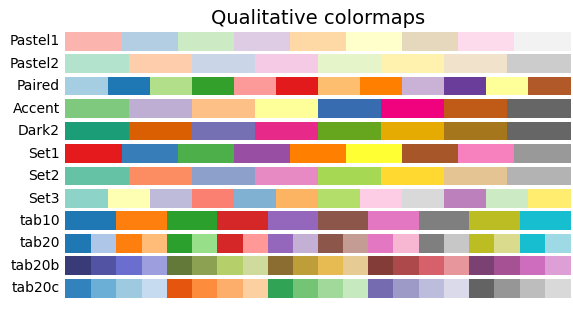In : cm.Set1.colors
Out:
((0.8941176470588236, 0.10196078431372549, 0.10980392156862745),
(0.21568627450980393, 0.49411764705882355, 0.7215686274509804),
(0.30196078431372547, 0.6862745098039216, 0.2901960784313726),
(0.596078431372549, 0.3058823529411765, 0.6392156862745098),
(1.0, 0.4980392156862745, 0.0),
(1.0, 1.0, 0.2),
(0.6509803921568628, 0.33725490196078434, 0.1568627450980392),
(0.9686274509803922, 0.5058823529411764, 0.7490196078431373),
(0.6, 0.6, 0.6))

In : cm.Set1.N
Out: 9


cmap 对象可以直接用数值参数调用，索引数值对应的 RGBA 值。根据数值是整型还是浮点型，对应关系也会有所不同，如下图所示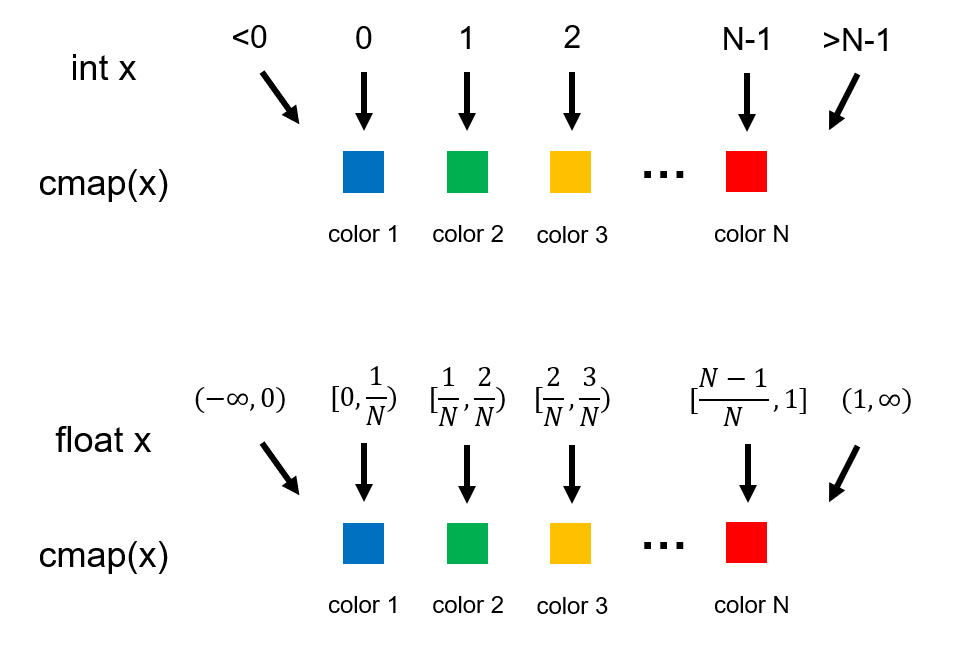In : cmap(np.arange(cmap.N))
Out:
array([[1.        , 0.54901961, 0.        , 1.        ],
[1.        , 0.84313725, 0.        , 1.        ],
[0.48627451, 0.98823529, 0.        , 1.        ],
[0.1254902 , 0.69803922, 0.66666667, 1.        ]])

In : cmap(np.linspace(0, 1, cmap.N))
Out:
array([[1.        , 0.54901961, 0.        , 1.        ],
[1.        , 0.84313725, 0.        , 1.        ],
[0.48627451, 0.98823529, 0.        , 1.        ],
[0.1254902 , 0.69803922, 0.66666667, 1.        ]])


cmap_new = mcolors.ListedColormap(
cmap(np.linspace(0, 1, 5))
)
show_cmap(cmap_new)


cmap_new 看起来会是这个样子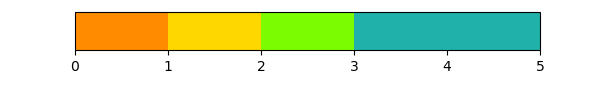### 1.2 LinearSegmentedColormap

LinearSegmentedColormap.from_list(name, colors, N=256, gamma=1.0)


name 是对象的名字，这回躲不掉必须填了；colors 是锚点的颜色，锚点对应的数值默认等距分布在 [0, 1] 区间上，不过可以在 colors 的每个颜色前指定数值；N 指定最后插值出几个颜色，默认为 256，所以基本看不出颜色间的间隔；gamma 是伽马校正的参数。例如

cmap1 = mcolors.LinearSegmentedColormap.from_list('cmap1', colors)
show_cmap(cmap1)

nodes = [0, 0.8, 0.9, 1]
cmap2 = mcolors.LinearSegmentedColormap.from_list(
'cmap2', list(zip(nodes, colors))
)
show_cmap(cmap2)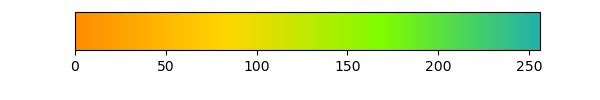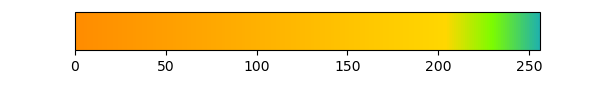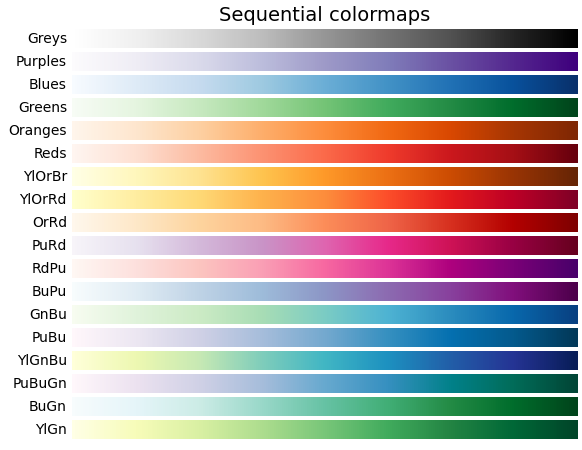In : cm.jet.colors
-------------------------------------------------------------------------
AttributeError: 'LinearSegmentedColormap' object has no attribute 'colors

In : cm.jet.N
Out: 256


LinearSegmentedColormap 虽然由 N 个颜色组成，但不能像 ListedColormap 那样把它们直接列举出来。cmap 同样可以被调用，当参数 x 为整数时，对应于第 x + 1 个颜色；当 x 为浮点数时，会通过线性插值获取相邻两个颜色中间的颜色。因此，LinearSegmentedColormap 的重采样不仅不会出现重复的颜色，还能得到更为连续渐变的颜色。不过有一说一，当颜色足够多时（即 N 很大时），两种 colormap 的区别就微乎其微了。

### 1.3 get_cmap 函数

# 等价于cm.jet(np.linspace(0, 1, 8))
cmap = cm.get_cmap('jet', 8)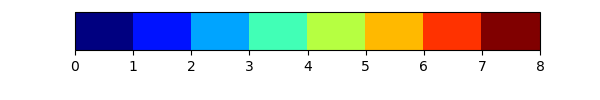1.1 节中提到过，直接调用 cmap 时，若参数 x 超出范围，那么会映射给第一个或最后一个颜色。而 cmapset_under 方法能够改变 x < 0 时对应的颜色，set_over 方法能够改变 x > N - 1x > 1 时对应的颜色。set_bad 则能改变缺测值对应的颜色（见 NumPy 系列：缺测值处理 最后一节）。

cmap = cm.get_cmap('jet', 8)
cmap.set_under('black')
cmap.set_over('white')
show_cmap(cmap, extend='both')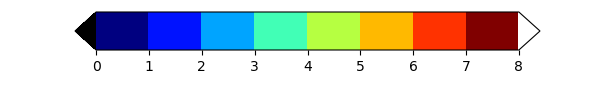### 1.5 修改内置 colormap

get_cmap 函数重采样得到的 colormap 可以直接用 set_xxx 系列方法进行修改，但对内置的 colormap 这样操作则会产生 MatplotlibDeprecationWarning。因为内置 colormap 都是全局对象，原地修改时会影响全局的效果。将来这一行为将会直接报错，官方建议先拷贝再修改。

import copy

cmap = copy.copy(cm.jet)
cmap.set_under('black')
cmap.set_over('white')


### 1.6 拼接内置 colormap

colors_cool = cm.cool(np.linspace(0, 1, 128))
colors_spring = cm.spring(np.linspace(0, 1, 128))
colors_all = np.vstack((colors_cool, colors_spring))
cmap_merged = mcolors.ListedColormap(colors_all)
show_cmap(cmap_merged)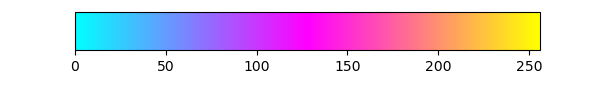## 2. Normalization

### 2.1 Normalize

Normalize(vmin=None, vmax=None, clip=False)


x = np.arange(0, 6)
norm = mcolors.Normalize()

In : norm(x)
Out:
masked_array(data=[0. , 0.2, 0.4, 0.6, 0.8, 1. ],
fill_value=1e+20)


norm 归一化后的值可以传给 colormap，进而按第一节介绍的映射规则得到画图用的颜色。即便 y 超出了 [0, 1] 的范围，也可以映射给第一个或最后一个颜色（或者 set_underset_over 指定的颜色）。换句话说，[vmin, vmax] 范围外的 x 自然对应于 colormap 两端的颜色。

clip 参数为 True 时，能把 [vmin, vmax] 范围外的 x 映射为 0 或 1，因此使 set_underset_over 的设置失效。一般默认为 False 即可。

### 2.2 LogNorm

LogNorm 的参数与 Normalize 相同，会先对数据求对数后再进行线性映射 $$y = \frac{\log_{10}(x) - \log_{10}(vmin)}{\log_{10}(vmax) - \log_{10}(vmin)}$$ 其中 vminvmax 必须为正数，否则会报错；x 可以小于等于 0，不过结果会缺测。例如

x = np.logspace(0, 3, 6)
norm = mcolors.LogNorm(vmin=1E0, vmax=1E3)

In : norm(x)
Out:
masked_array(data=[0.0, 0.2, 0.4, 0.6, 0.8, 1.0],
mask=[False, False, False, False, False, False],
fill_value=1e+20)


### 2.3 TwoSlopeNorm

[vmin, vmax] 分成两个区间，进行分段线性映射。参数为

TwoSlopeNorm(vcenter, vmin=None, vmax=None)


### 2.4 BoundaryNorm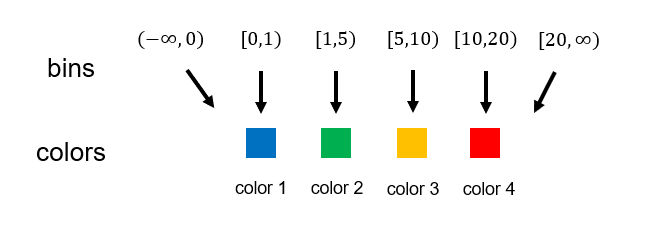BoundaryNorm(boundaries, ncolors, clip=False, extend='neither')


boundaries 为给出的这些 bin 的边缘数值，要求单调递增；ncolors 指定对应的 colormap 含有的颜色数，要求数值大于等于 nbin = len(boundaries) - 1。当 ncolors = nbin 时，映射关系为 $$y = \begin{cases} -1 &\text{if} \quad x < boundaries \newline i &\text{if} \quad boundaries[i] \le x < boundaries[i+1] \newline nbin &\text{if} \quad x \ge boundaries[-1] \end{cases}$$ 可以看到，落入框中的 x 会被映射到 [0, nbin - 1] 区间，而没有落入框中的 x 会映射为 -1 或 nbin。当 ncolors > nbin 时，程序会通过线性插值（并取整）把上面的结果再映射到 [0, ncolors - 1] 区间。所以可以有两种使用方法：

• ncolorscmap.N，配合完整的 colormap。
• ncolorsnbin，再把 colormap 采样到只含 nbin 个颜色。

extend 参数会增大 nbin 以改变映射结果，直观效果是使第一个和最后一个 bin 对应的颜色区别于 under 和 over 时的颜色。考虑到会使映射关系变复杂，所以我一般不会去设置，但对该效果有需求的读者可以自己试试。下面是一个简单例子

bins = [0, 0.1, 0.5, 1.0, 5.0, 10.0]
nbin = len(bins) - 1
norm = mcolors.BoundaryNorm(bins, nbin)
x = [-1, 0.05, 0.2, 0.6, 2, 8, 12]

In : norm(x)
Out:
masked_array(data=[-1, 0, 1, 2, 3, 4, 5],
mask=[False, False, False, False, False, False, False],
fill_value=999999,
dtype=int64)


## 3 实际应用

### 3.1 pcolor 和 contour 的异同

# 生成测试数据.
x = np.linspace(0, 10, 100)
y = np.linspace(0, 10, 100)
X, Y = np.meshgrid(x, y)
Z = 1E3 * np.exp(-(np.abs(X - 5)**2 + np.abs(Y - 5)**2))

fig, axes = plt.subplots(1, 2, figsize=(12, 5))

# 直接给出vmin和vmax时会自动用它们创建Normalize.
im = axes.pcolormesh(
cmap=cm.jet, vmin=0, vmax=1000
)
cbar = fig.colorbar(im, ax=axes, extend='both')
axes.set_title('Normalize')

# 若在pcolormesh中给定了norm,就不能再指定vmin和vmax了.
im = axes.pcolormesh(
cmap=cm.jet, norm=mcolors.LogNorm(vmin=1E-3, vmax=1E3)
)
# 使用LogNorm时,colorbar会自动选用_ColorbarLogLocator来设定刻度.
cbar = fig.colorbar(im, ax=axes, extend='both')
axes.set_title('LogNorm')

plt.show()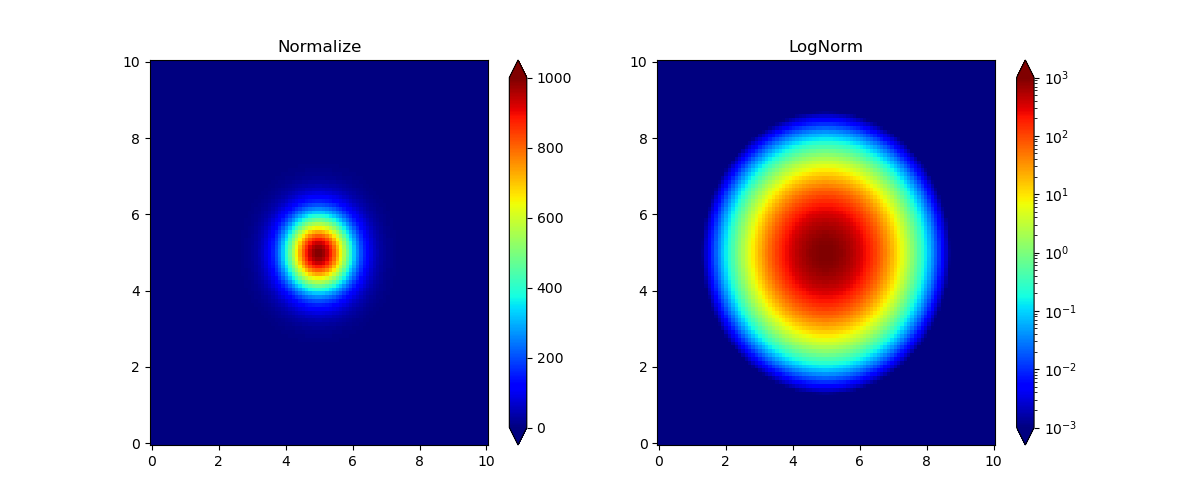# 在norm不是LogNorm的情况下,layers计算为levels的中点.详请参考matplotlib.contour模块.
levels = np.asarray(levels)
layers = 0.5 * (levels[1:] + levels[:-1])
colors = cmap(norm(layers))


contourf 默认不会填充 levels 范围以外的颜色，如果有这方面的需求，可以用 extend 参数指定是否让超出范围的数据被填上 colormap 两端的颜色（或 set_underset_over 指定的颜色）。并且当 contourf 指定了 extend 后，就不要在 colorbar 里指定了，否则会产生警告乃至错误。

# 生成测试数据.
x = np.linspace(0, 10, 100)
y = np.linspace(0, 10, 100)
X, Y = np.meshgrid(x, y)
Z = (X - 5) ** 2 + (Y - 5) ** 2
# 将Z的值缩放到[0, 100]内.
Z = Z / Z.max() * 100

# 设置一个简单的colormap.
cmap = mcolors.ListedColormap(['blue', 'orange', 'red', 'purple'])
fig, ax = plt.subplots()
# contour和contourf默认使用levels的最小最大值作为vmin和vmax.
levels = np.linspace(10, 60, 6)
im1 = ax.contourf(X, Y, Z, levels=levels, cmap=cmap, alpha=0.5)
im2 = ax.contour(X, Y, Z, levels=levels, cmap=cmap, linewidths=2)
cbar = fig.colorbar(im1, ax=ax, ticks=levels)
# 为等高线添加标签.
ax.clabel(im2, colors='k')

plt.show()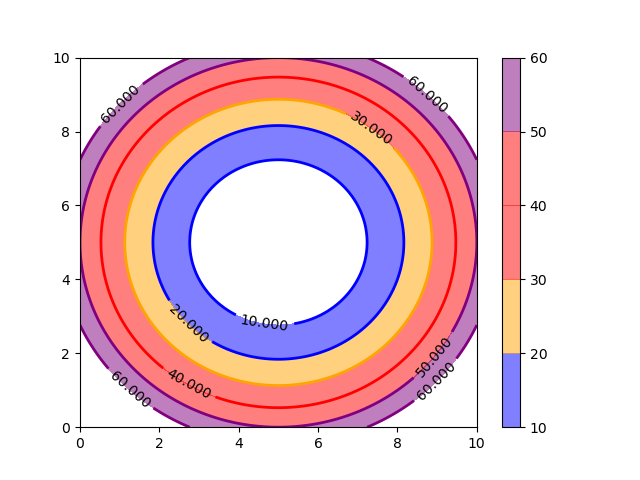### 3.2 BoundaryNorm 的应用

# 生成测试数据.
x = np.linspace(0, 10, 100)
y = np.linspace(0, 10, 100)
X, Y = np.meshgrid(x, y)
Z = X ** 2 + Y ** 2
# 将Z的值缩放到[0, 100]内.
Z = Z / Z.max() * 100

# 设置norm.
bins = [1, 5, 10, 20, 40, 80]
nbin = len(bins) - 1
norm = mcolors.BoundaryNorm(bins, nbin)
# 设置cmap.
cmap = cm.get_cmap('jet', nbin)
cmap.set_under('white')
cmap.set_over('purple')

fig, axes = plt.subplots(1, 2, figsize=(12, 5))

# 使用pcolormesh.
im = axes.pcolormesh(X, Y, Z, cmap=cmap, norm=norm, shading='nearest')
cbar = fig.colorbar(im, ax=axes, ticks=bins, extend='both')
axes.set_title('pcolormesh')

# 使用contourf.
im = axes.contourf(
X, Y, Z, levels=bins, cmap=cmap, norm=norm, extend='both'
)
cbar = fig.colorbar(im, ax=axes, ticks=bins)
axes.set_title('contourf')

plt.show()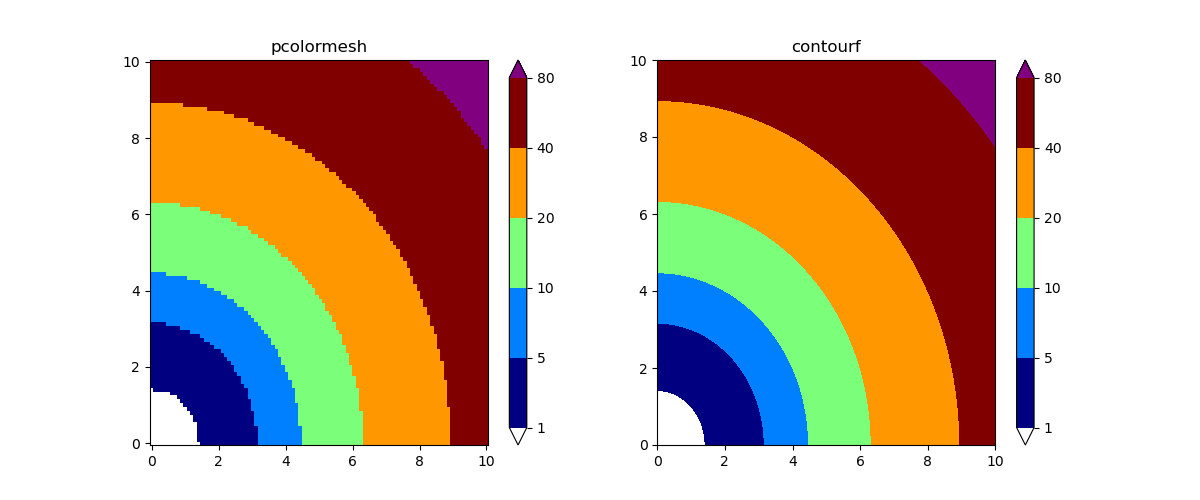### 3.3 红蓝 colormap

Normalize(vmin=-10, vmax=10)


TwoSlopeNorm(vmin=-5, vcenter=0, vmax=10)


class CenteredBoundaryNorm(mcolors.BoundaryNorm):
'''将vcenter所在的bin映射到cmap中间的BoundaryNorm.'''
def __init__(self, boundaries, vcenter=0, clip=None):
super().__init__(boundaries, len(boundaries) - 1, clip)
boundaries = np.asarray(boundaries)
self.N1 = np.count_nonzero(boundaries < vcenter)
self.N2 = np.count_nonzero(boundaries > vcenter)
if self.N1 < 1 or self.N2 < 1:
raise ValueError('vcenter两侧至少各有一条边界')

def __call__(self, value, clip=None):
# 将BoundaryNorm的[0, N-1]又映射到[0.0, 1.0]内.
result = super().__call__(value, clip)
if self.N1 + self.N2 == self.N - 1:
result = np.ma.where(
result < self.N1,
result / (2 * self.N1),
(result - self.N1 + self.N2 + 1) / (2 * self.N2)
)
else:
result = np.ma.where(
result < self.N1,
result / (2 * (self.N1 - 1)),
(result - self.N1 + self.N2) / (2 * (self.N2 - 1))
)

return result


# 生成测试数据.
X, Y = np.meshgrid(np.linspace(-2, 2, 100), np.linspace(-2, 2, 100))
Z1 = np.exp(-X**2 - Y**2)
Z2 = np.exp(-(X - 1)**2 - (Y - 1)**2)
Z = ((Z1 - Z2) * 2)
# 将Z的值缩放到[-5, 10]内.
Z = (Z - Z.min()) / (Z.max() - Z.min()) * 15 - 5

fig, axes = plt.subplots(1, 2, figsize=(12, 5))

# TwoSlopeNorm的图.
cf = axes.contourf(
X, Y, Z,
levels=np.linspace(-5, 10, 16),
cmap='RdBu_r',
norm=mcolors.TwoSlopeNorm(vmin=-5, vcenter=0, vmax=10),
extend='both'
)
cbar = fig.colorbar(cf, ax=axes, ticks=cf.levels)
axes.set_title('TwoSlopeNorm')

# BoundaryNorm的图.
boundaries = [-5, -3, -2, -1, -0.1, 0.1, 1, 2, 4, 6, 8, 10]
im = axes.contourf(
X, Y, Z,
levels=boundaries,
cmap='RdBu_r',
norm=CenteredBoundaryNorm(boundaries),
extend='both'
)
cbar = fig.colorbar(im, ax=axes, ticks=boundaries)
axes.set_title('BoundaryNorm')

plt.show()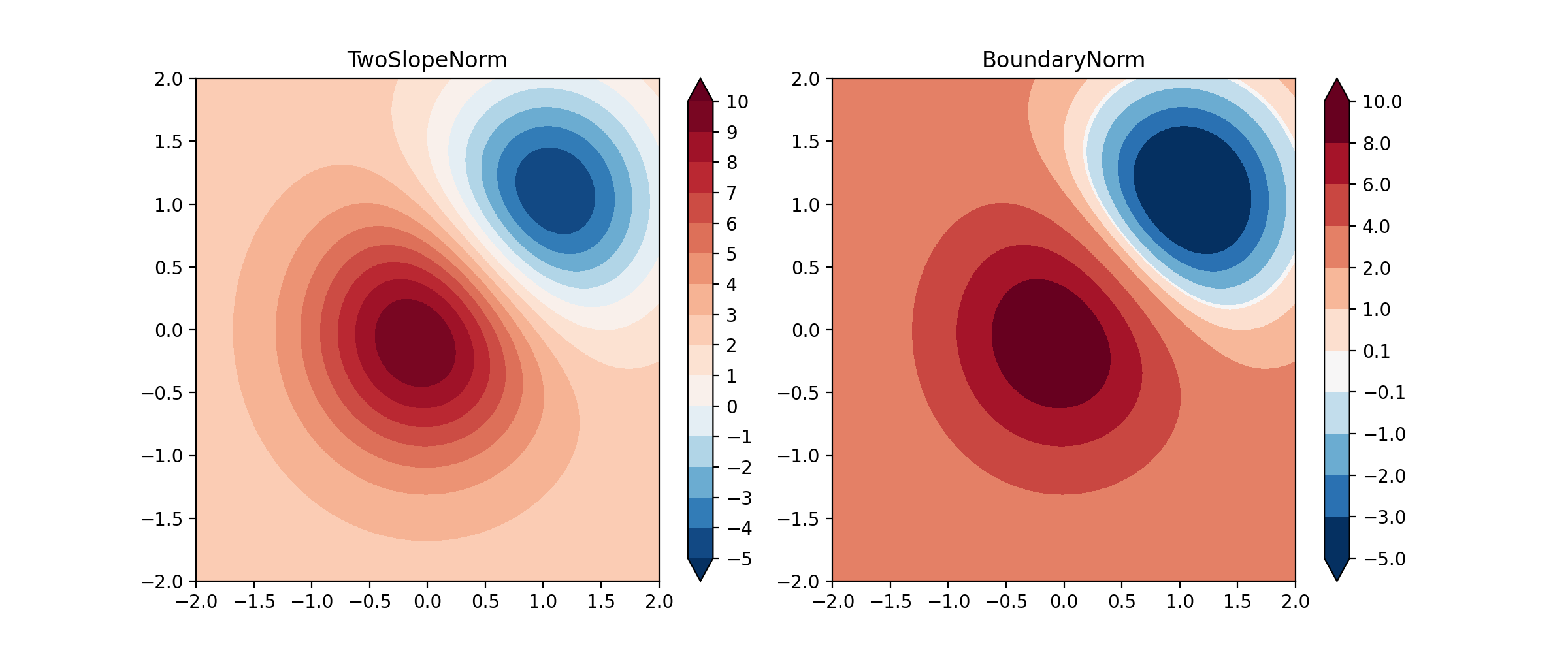## 参考链接

Customized Colorbars Tutorial

Creating Colormaps in Matplotlib

Colormap Normalization

Beautiful custom colormaps with Matplotlib